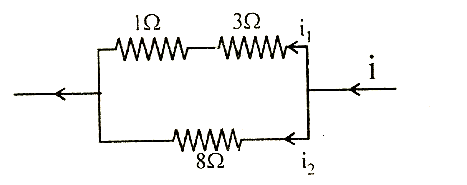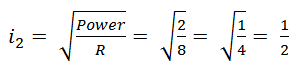# Power dissipated across the 80 resistor in the circuit shown here is 2 watt. The power dissipated in watt units across the 30 resistor is(a)    1.0         (b)    0.5         (c)    3.0         (d)    2.0Aditi Chauhan
10 years ago

(c)

Power = V. I = 12RPotential over 80 = Ri2 = 8 ×0.5  = 4V

This is the potential over parallel branch.

So,

Power of 3W = i1\2R = 1 × 1 × 3 = 3W